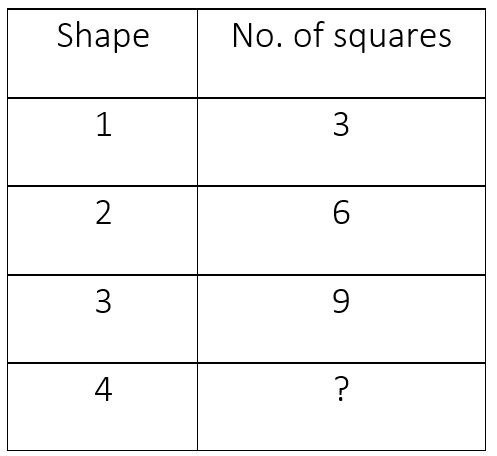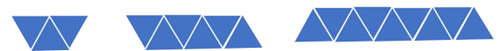General
General
Easy

Question

# John creates an increasing pattern with triangles. He records the number of triangles in each shape in a table of values. He also records the number of triangles he adds each time he makes a new shape.## 10    12    15    14Hint:

## The correct answer is: 12

### The number of shape and number of triangles used to make the shape are as follows:First shape has 3 triangles.Second shape has 6 triangles.Third shape has 9 triangles.If we see, the number of triangles used is increasing by 3. With every shape, the number is increasing by 3.So, fourth shape will have (9 + 3) = 12 triangles.The fourth shape will have 12 triangles.

While solving such questions, we should be careful and the sequence.

### Related Questions to study#### With Turito Foundation.#### Get an Expert Advice From Turito.